# Saliency Maps的原理与简单实现(使用Pytorch实现)

• A+

## Saliency Maps的原理

### 简单解释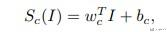### 对于复杂的网络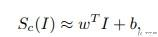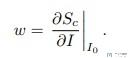### Saliency Maps的另外一种解释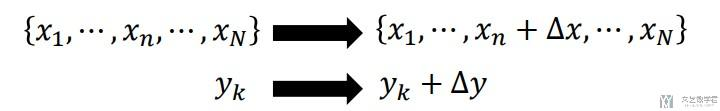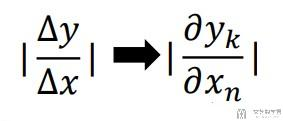### Saliency Maps实验结果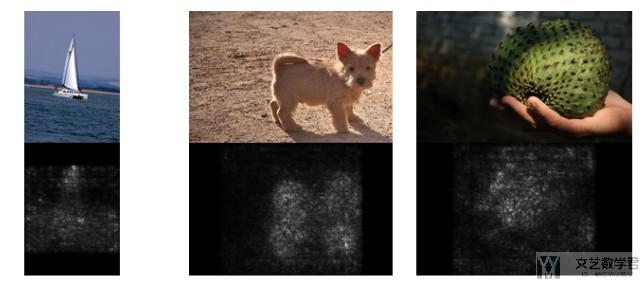## Saliency Maps的具体操作

• 计算Saliency Map的时候首先要计算与图像像素对应的正确分类中的标准化分数的梯度(这是一个标量)。如果图像的形状是(3, H, W)，这个梯度的形状也是(3, H, W)；
• 对于图像中的每个像素点，这个梯度告诉我们当像素点发生轻微改变时，正确分类分数变化的幅度。
• 之后，我们计算出梯度的绝对值，然后再取三个颜色通道的最大值；因此最后的saliency map的形状是(H, W)为一个通道的灰度图。

## Saliency Maps简单实现(Pytorch版本)

### 计算输入X的导数

1. def compute_saliency_maps(X, y, model):
2.     """
3.     X表示图片, y表示分类结果, model表示使用的分类模型
4.
5.     Input :
6.     - X : Input images : Tensor of shape (N, 3, H, W)
7.     - y : Label for X : LongTensor of shape (N,)
8.     - model : A pretrained CNN that will be used to computer the saliency map
9.
10.     Return :
11.     - saliency : A Tensor of shape (N, H, W) giving the saliency maps for the input images
12.     """
13.     # 确保model是test模式
14.     model.eval()
17.     saliency = None
18.     logits = model.forward(X)
19.     logits = logits.gather(1, y.view(-1, 1)).squeeze() # 得到正确分类
20.     logits.backward(torch.FloatTensor([1., 1., 1., 1., 1.])) # 只计算正确分类部分的loss
21.     saliency = abs(X.grad.data) # 返回X的梯度绝对值大小
22.     saliency, _ = torch.max(saliency, dim=1)
23.     return saliency.squeeze()

### 显示实验结果

1. def show_saliency_maps(X, y):
2.     # Convert X and y from numpy arrays to Torch Tensors
3.     X_tensor = torch.cat([preprocess(Image.fromarray(x)) for x in X], dim=0)
4.     y_tensor = torch.LongTensor(y)
5.     # Compute saliency maps for images in X
6.     saliency = compute_saliency_maps(X_tensor, y_tensor, model)
7.     # Convert the saliency map from Torch Tensor to numpy array and show images
8.     # and saliency maps together.
9.     saliency = saliency.numpy()
10.     N = X.shape
11.     for i in range(N):
12.         plt.subplot(2, N, i + 1)
13.         plt.imshow(X[i])
14.         plt.axis('off')
15.         plt.title(class_names[y[i]])
16.         plt.subplot(2, N, N + i + 1)
17.         plt.imshow(saliency[i], cmap=plt.cm.hot)
18.         plt.axis('off')
19.         plt.gcf().set_size_inches(12, 5)
20.     plt.show()
21. show_saliency_maps(X, y)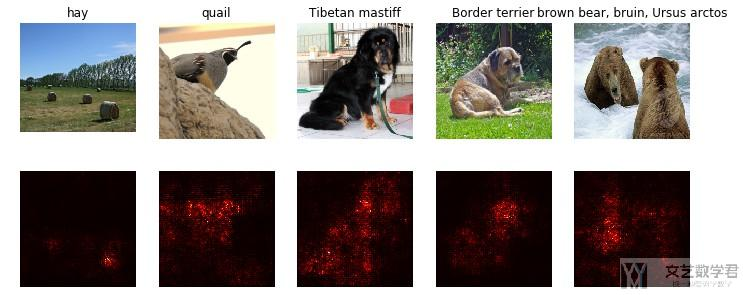### 关于gather的一些说明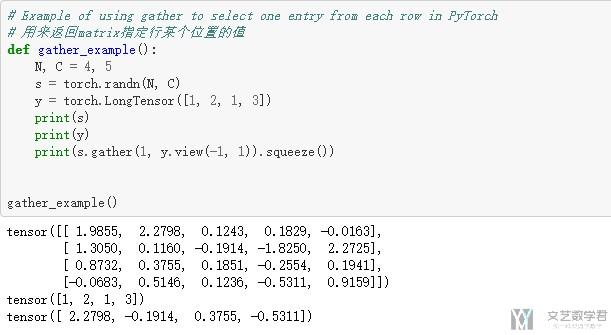## Saliency Maps的应用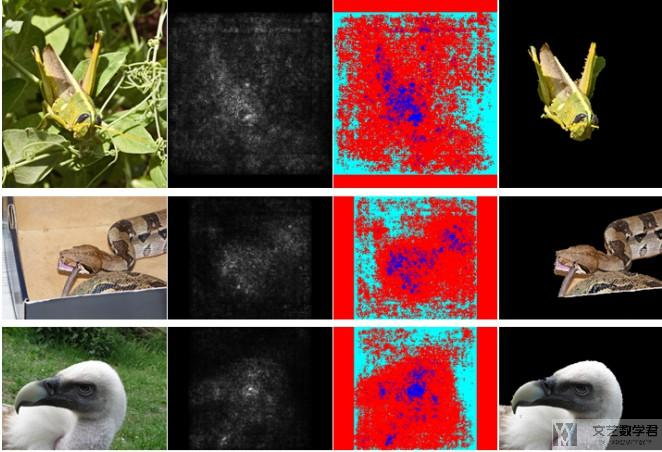• 最左侧为原图像；
• 第二列表示计算得到的Saliency Maps；
• 第三列表示作者设定了一个阈值，超过认为是重要的(图中蓝色的部分是比较重要的)；
••